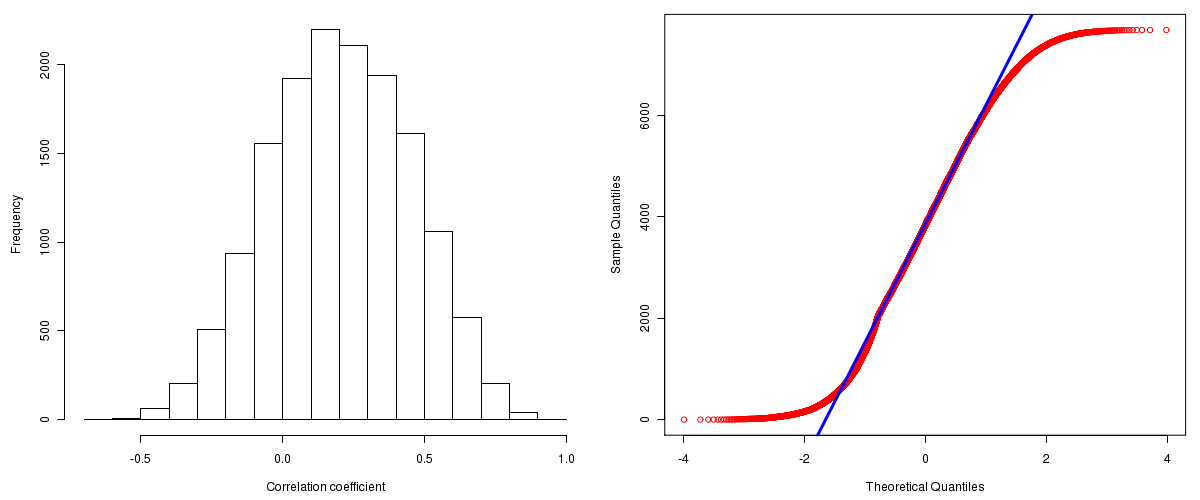Lung Adenocarcinoma: Correlations between copy number and mRNA expression
Maintained by John Zhang (Dana-Farber Cancer Institute)
Overview
Introduction

A TCGA sample is profiled to detect the copy number variations and expressions of genes. This pipeline attempts to correlate copy number and expression data of genes across samples to determine if the copy number variations also result in differential expressions. This report contains the calculated correlation coefficients based on measurements of genomic copy number (log2) values and intensity of the expressions of the corresponding feature across patients. High positive/low negative correlation coefficients indicate that genomic alterations result in differences in the expressions of mRNA the genomic regions transcribe.

Summary

The correlation coefficients in 10, 20, 30, 40, 50, 60, 70, 80, 90 percentiles are -0.11866, -0.0159, 0.0651, 0.13574, 0.2039, 0.2728, 0.34674, 0.4259, 0.53034, respectively.

Results
Correlation results

Number of genes and samples used for the calculation are shown in Table 1. Figure 1 shows the distribution of calculated correlation coefficients and quantile-quantile plot of the calculated correlation coefficients against a normal distribution. Table 2 shows the top 20 features ordered by the value of correlation coefficients.

Table 1.  Counts of mRNA and number of samples in copy number and expression data sets and common to both

Category Copy number Expression Common
Sample 205 32 32
Genes 29390 17815 15551

Figure 1.  Summary figures. Left: histogram showing the distribution of the calculated correlations across samples for all Genes. Right: QQ plot of the calculated correlations across samples. The QQ plot is used to plot the quantiles of the calculated correlation coefficients against that derived from a normal distribution. Points deviating from the blue line indicate deviation from normality.Table 2.  Get Full Table Top 20 features (defined by the feature column) ranked by correlation coefficients

feature r p-value q-value chrom start end geneid
C14orf166 0.9164 1.83408843668076e-13 1.48606506585004e-09 14 51525943 51541163 51637
PIK3C3 0.8947 5.04929431599521e-12 2.04558835335532e-08 18 37789197 37915446 5289
PSMC6 0.8755 5.49473799793532e-11 1.21251035245718e-07 14 52243668 52264466 5706
SCFD1 0.8747 5.985878459569e-11 1.21251035245718e-07 14 30161272 30274769 23256
PSMA6 0.8719 8.15387757313601e-11 1.24272341057563e-07 14 34831325 34856431 5687
FBXO33 0.8708 9.20254983327595e-11 1.24272341057563e-07 14 38936710 38971371 254170
FKBP3 0.864 1.90520044185405e-10 2.20526195813048e-07 14 44654859 44674272 2287
ORAOV1 0.8536 5.32451416290769e-10 5.24106003139271e-07 11 69189512 69199346 220064
MOCS3 0.8517 6.4022964707533e-10 5.24106003139271e-07 20 49008770 49011227 27304
C18orf21 0.8516 6.46847020391306e-10 5.24106003139271e-07 18 31806586 31813239 83608
MRPL21 0.8498 7.61579244112909e-10 5.60970426367557e-07 11 68415322 68427879 219927
PAPD1 0.8476 9.29671895022466e-10 5.97327022420323e-07 10 30641765 30678254 55149
ZNF24 0.8473 9.58380486082433e-10 5.97327022420323e-07 18 31169957 31178405 7572
LSG1 0.836 2.5887350041387e-09 1.47696288733654e-06 3 195842812 195874200 55341
SMAD2 0.8353 2.73428191199798e-09 1.47696288733654e-06 18 43618435 43711221 4087
C14orf28 0.8335 3.18995252435172e-09 1.61147892472239e-06 14 44436257 44446210 122525
SRP54 0.8328 3.3810843014237e-09 1.61147892472239e-06 14 34521982 34568519 6729
DNM1L 0.8296 4.38610658903826e-09 1.9743503841252e-06 12 32723491 32788621 10059
UBL7 0.828 4.97449881287082e-09 2.12135466204048e-06 15 72525371 72540582 84993
MRPL51 0.825 6.35796704173686e-09 2.42586096762293e-06 12 6471577 6472718 51258
Methods & Data
Input

Gene level (TCGA Level III) expression data and copy number data of the corresponding loci derived by using the CNTools package of Bioconductor were used for the calculations. Pearson correlation coefficients were calculated for each pair of genes shared by the two data sets across all the samples that were common.

Correlation across sample

Pairwise correlations between the log2 copy numbers and expressions of each gene across samples were calculated using Pearson correlation.